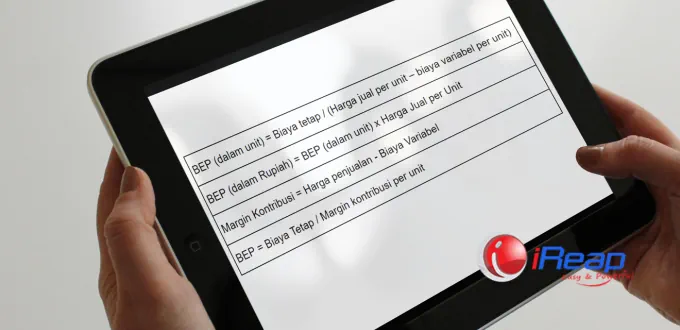# How to Calculate BEP (Break Event Point)BEP is a breakeven point that represents the balance between the number of products sold and the cost of producing those products. There are two types of BEP calculations: unit BEP and BEP in rupiah. Learn in detail the components of BEP and how to calculate BEP in this article, let’s go.

## 4 Components of BEP (Break-Even Point)

Why is it necessary to calculate BEP in running a business? Because a detailed Break-Even Point (BEP) calculation will help you identify the sales target that needs to be achieved to make a profit.

Now, before calculating BEP, get to know the 4 components of BEP:

### 1. Fixed Costs

Fixed costs are expenses that remain unchanged regardless of the sales volume generated.

Even when the production volume changes, these costs will remain constant. Examples of fixed costs include rent, salaries of permanent employees, insurance, and others.

### 2. Variable Costs

Variable costs are expenses that change with the changes in the production volume.

Examples of variable costs include raw material costs, labor wages that fluctuate due to overtime, and other production costs.

### 3. Selling Price Per Unit

The selling price per unit is the revenue the company obtains from selling one unit of product or service.

### 4. Total Units Sold

Total units sold refer to the total number of units of products or services sold within a specific period.## Methods for Calculating BEP

There are 3 methods you can use to calculate BEP:

### 1. Marketing Method

Using the marketing method requires financial data in the form of profit and loss reports.

### 2. Unit Contribution Method

On the other hand, the unit contribution method is calculated based on the total contribution margin. The contribution margin is the difference between the total revenue from sales and the variable costs.

### 3. Graph Method

The third method is the graph method. In this method, BEP is illustrated in the form of a graph.

Each of these three methods has its advantages and disadvantages. As a business practitioner, it is essential to understand the basics of these methods.

## BEP Formula

The simple formula for calculating the Break-Even Point is as follows:## How to Calculate BEP

Take a look at the BEP formula above. Then, read the example problem below to understand how to calculate BEP:

A company has fixed production costs of Rp50,000,000. It is also known that the variable cost per unit is Rp30,000, and the selling price per unit is Rp50,000. The company has a profit target of Rp20,000,000.

Now, to determine how many units need to be produced, use the BEP formula and the contribution margin formula.

BEP = Fixed Costs / (Contribution Margin Formula)

BEP = Fixed costs / (selling price per unit – variable cost per unit)
BEP = 50,000,000 / (50,000 – 30,000)
BEP = 2,500 units

So, with the fixed cost of 50 million Rupiah, the company will only break even if it produces 2,500 units and sells all of them.

To calculate the BEP in rupiah, use the following BEP formula:

BEP (in Rupiah) = BEP (in units) x Selling Price per Unit
BEP (in Rupiah) = 50,000 x 2,500 units
BEP (in Rupiah) = Rp125,000,000

By understanding how to calculate BEP (break-even point), the components of BEP, and the BEP formula, you can determine the appropriate selling price for the business to achieve. Oh, if you have a well-organized inventory and stock management, you can also calculate the selling price in another way. Learn how to do it here: Easy Way to Calculate Cost of Goods Sold (COGS) & Selling Price.